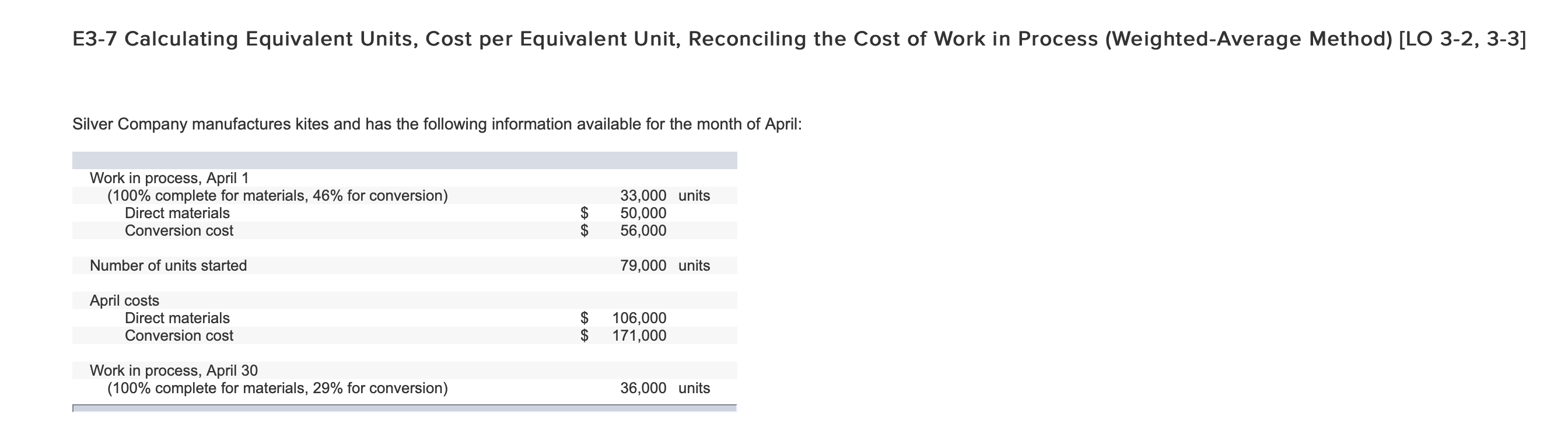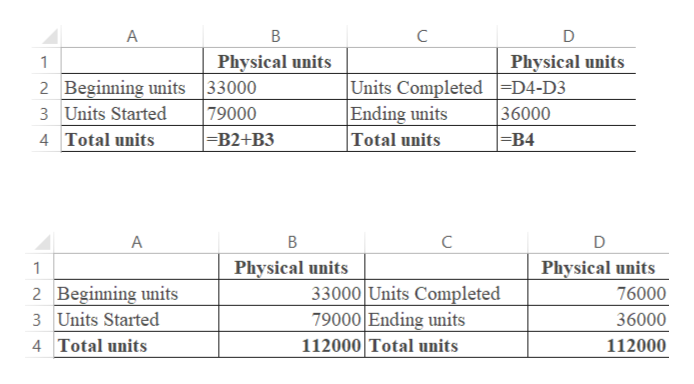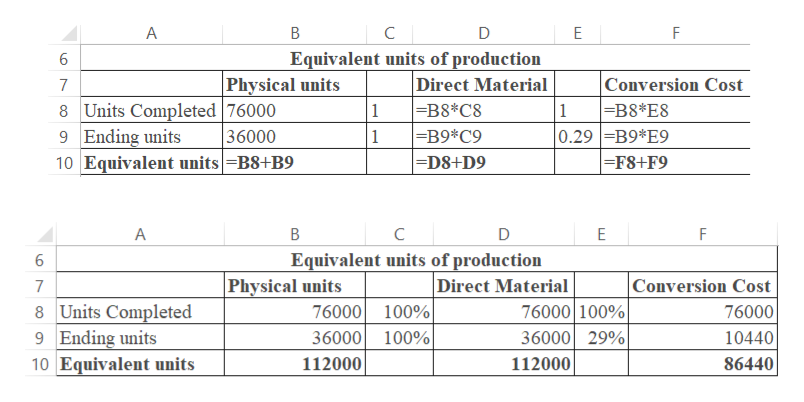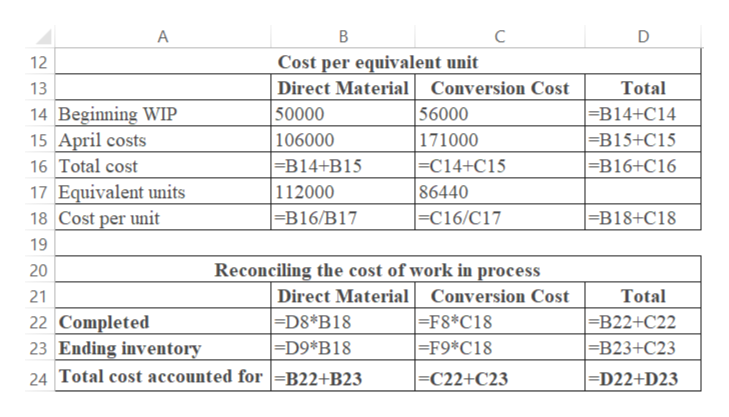# E3-7 Calculating Equivalent Units, Cost per Equivalent Unit, Reconciling the Cost of Work in Process (Weighted-Average Method) [LO 3-2, 3-3]Silver Company manufactures kites and has the following information available for the month of April:Work in process, April 1(100% complete for materials, 46% for conversion)Direct materials33,000 units50,00056,000Conversion cost\$Number of units started79,000 unitsApril costsDirect materials106,000171,000Conversion costWork in process, April 30(100% complete for materials, 29% for conversion)36,000 unitsEA CA

Question
87 views

1. reconcile the number of physical units

2.calculate the number of equivalent units

3.calculate the cost per unit

4. record the total cost of work in process

I am unsure how to do this problem and keep getting it incorrecthelp_outlineImage TranscriptioncloseE3-7 Calculating Equivalent Units, Cost per Equivalent Unit, Reconciling the Cost of Work in Process (Weighted-Average Method) [LO 3-2, 3-3] Silver Company manufactures kites and has the following information available for the month of April: Work in process, April 1 (100% complete for materials, 46% for conversion) Direct materials 33,000 units 50,000 56,000 Conversion cost \$ Number of units started 79,000 units April costs Direct materials 106,000 171,000 Conversion cost Work in process, April 30 (100% complete for materials, 29% for conversion) 36,000 units EA CA fullscreen
check_circle

Step 1

1.

Reconciling the number of physical units:

The results of the formulas used in the excel sheet are shown in the following table:help_outlineImage TranscriptioncloseC A Physical units Physical units 2 Beginning units |33000 79000 =B2+B3 Units Completed -D4-D3 Ending units Total units 36000 =B4 3 Units Started 4 Total units A B C Physical units Physical units 1 33000 Units Completed 79000 Ending units 112000 Total units 2 Beginning units 3 Units Started 76000 36000 4 Total units 112000 fullscreen
Step 2

2

Calculation of number of equivalent units:

The results of the formulas used in the excel sheet are shown in the following table:help_outlineImage TranscriptioncloseA В C D E Equivalent units of production Direct Material =B8*C8 |=B9*C9 -D8+D9 6 Physical units Conversion Cost =B8*E8 0.29 B9*E9 -F8+F9 7 8 Units Completed |76000 1 1 36000 9 Ending units 1 10 Equivalent units|=B8+B9 A В C F Equivalent units of production 6 |Physical units Direct Material 76000 100% 36000 29% Conversion Cost 7 76000 100% 36000 100% 8 Units Completed 9 Ending units 10 Equivalent units 76000 10440 112000 112000 86440 fullscreen
Step 3
1. Calculation of cost per unit...help_outlineImage TranscriptioncloseA B C Cost per equivalent unit Direct Material 50000 106000 -B14+B15 112000 |=B16/B17 12 13 Conversion Cost Total =B14+C14 =B15+C15 =B16+C16 56000 171000 =C14+C15 86440 -C16/C17 14 Beginning WIP 15 April costs 16 Total cost 17 Equivalent units 18 Cost per unit =B18+C18 19 Reconciling the cost of work in process Direct Material =D8*B18 |=D9*B18 20 Conversion Cost Total 21 =F8*C18 =F9*C18 =B22+C22 |=B23+C23 22 Completed 23 Ending inventory 24 Total cost accounted for =B22+B23 =D22+D23 |=C22+C23 fullscreen

### Want to see the full answer?

See Solution

#### Want to see this answer and more?

Solutions are written by subject experts who are available 24/7. Questions are typically answered within 1 hour.*

See Solution
*Response times may vary by subject and question.
Tagged in